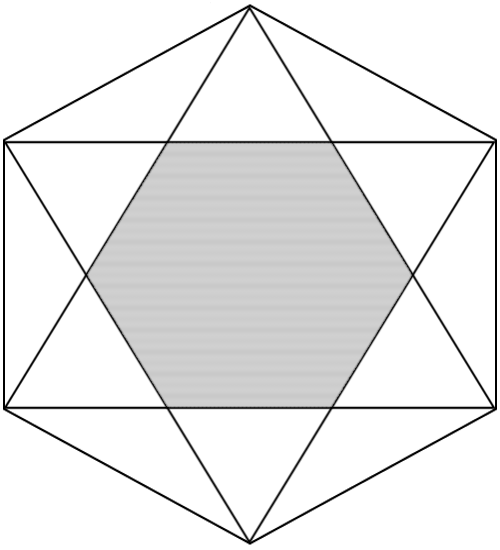# Horrible Hexagons 1

Geometry Level 3The diagram above shows a regular hexagon ${ H }_{ 1 }$ with two equilateral triangles inscribed in it.

If the shaded region has area $x$ $\text{ unit}^{ 2 }$, what is the area of ${ H }_{ 1 }$ in terms of $x$?

×

Problem Loading...

Note Loading...

Set Loading...